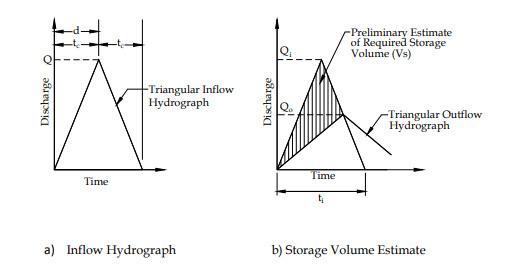603 - 5885 1250 (4 lines) info@mes100.com
Select Page

# Preliminary Pond Volume of A Pond

One of the final steps in designing a pond is to determine a suitable basin size that will be able to hold the  stormwater of a catchment temporarily before gradually releasing it to the water bodies. The stormwater will be released by the designed release rate (through the outlet design) to prevent erosion and flooding downstream.

## How is basin size determined? #

Designing a basin size requires input such as top length, top width, depth of pond, and the pond invert level from an engineer.

Based on these inputs, a stage vs storage curve will be developed, using the Double-End Area method [MSMA 2nd Edition, Chapter 7, Section 7.5.2]. This curve will give us the information on the storage level at each elevational stage (at every 0.05m), hence cumulatively calculates the volume able to be provided by the designed basin.

Question is, how do the engineers or the program estimate basin size are able to cater for the catchment area, before they go to the outlet design?

The answer to this is the preliminary pond volume storage of the catchment area, Vs.

## So, what is a preliminary pond volume? #

A preliminary pond volume is an estimation of how much stormwater a pond or a basin should hold for a catchment temporarily, especially during the rainy season.

Hence, this estimation of the required storage capacity should be obtained together with the storage facility shape beforehand.

This is because the suitability of the basin size will be determined by comparing the provided volume with the preliminary volume – the provided pond volume should be larger from the preliminary pond volume – while maintaining the provided pond top area to be between 3-5%.

## Now, how is preliminary pond volume computed? #

The estimation of pond storage, is determined for the Major Design Storm ARI using the Rational Method Hydrograph (RHM).

The storage volume estimated can be computed using the Equation 7.1 (both 7.1a and 7.1b, depending on the Hydrograph Type from MSMA 2nd Edition, Chapter 7.

Type 1 (7.1a): $$V_s=\;d\mathit\;Q_{\mathit i}\mathit-\mathit0\mathit.\mathit5\mathit\;t_{\mathit i}\mathit\;Q_{\mathit o}$$

Type 2 (7.1b) : $$V_s=\;\mathit0\mathit.\mathit5\mathit\;t_{\mathit i}\mathit\;\mathit{\left({Q_i-Q_o}\right)}$$

Where;

Vs = Volume storage (m3);

Qi = Peak Inflow (m3/s)

Qo = Peak Outflow (m3/s)

ti = Total duration of inflow; d + tc (sec)

Through RHM for both Type 1 and Type 2, the inflow hydrograph (Qinflow) – derived by the relationship of rainfall duration with respect to the time of concentration, tc, of the sub-catchment – is provided with the desired release rate (Qoutflow).

The difference between the Qinflow and Qoutlow (shaded area) obtained through the hydrograph will be taken as the preliminary estimate of the required storage volume.Estimation of the pond storage volume for Type 1 Hydrograph (Source: MSMA 2nd Edition, Chapter 7)Estimation of the pond storage volume for Type 2 Hydrograph (Source: MSMA 2 nd Edition, Chapter 7)

From the above equation, it can be inferred that the preliminary pond volume by itself doesn’t say much about the eventual suitable pond size, because the final pond size will depend critically on the discharge volume at outlet design, which preliminary pond volume doesn’t compute. It just serves as a preliminary number for you to work on.

## Benchmarking of the preliminary pond volume #

The calculation example based on the project here;

By applying Eq 7.1a;

\begin{aligned} ti &= d\mathit\;\mathit+\mathit\;t_{\mathit c}\ \\ t_i &= \left(34.5+13.80\right)\times60 \\ t_i &= 2898\;\text{seconds} \end{aligned}

\begin{aligned} Q_i &= 3.165\;m^3 \\ Q_o &= 2.193\;m^3 \end{aligned}

\begin{aligned} V_s &= d\;Q_i\;-\;\left(0.5\times t_i\times Q_o\right) \\ V_s &= \left(34.50\times60\times3.165\right)-\left(0.5\times2898\times2.193\right) \\ V_s &= 3373.893\;m^3 \end{aligned}

## Conclusion #

Take note that the actual pond size should be designed in conjunction with outlets. Thus prelim. Volume size is just a ballpark figure to aid engineers in the initial stage of volume estimation.